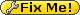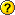# Optimizing bitslice DES S-box expressions

The algorithm consists of two major parts:

• breadth-first search of expressions for 5-bit inputs and 1-bit outputs
• depth-first search of 8 1-bit outputs

Represent the truth table of a logical function of 5 inputs as a 32-bit value `x`. Let complexity of `x`, which we'll denote with `C(x)`, be the minimum (or near-minimum) number of logical gates for a particular input.What is meant by “for a particular input”? Need to clarify. OKStandard inputs are: 0x55555555, 0x33333333, 0x0F0F0F0F, 0x00FF00FF, 0x0000FFFF. For these, `C(input)` is 0.

For example, complexity of 0x0F00F0FF is 2 for the SSE instruction set and standart inputs ( C(0x0F00F0FF)==2 ):

```0x0F000F00 = 0x0F0F0F0F & ~0x00FF00FF;
0x0F00F0FF = x0F000F00 ^ 0x0000FFFF;```

If We fix 0x0F00F0FF as result, We will get particular inputs: 0x55555555, 0x33333333, 0x0F0F0F0F, 0x00FF00FF, 0x0000FFFF, 0x0F000F00, 0x0F00F0FF

For these inputs C(0x0F000F00)==0 and C(0x0F00F0FF)==0.

In order to get all logical functions of complexity `n`, the algorithm must try:

• unary operations with logical functions of complexity i==n-1
• binary operations with logical functions of complexity i,j (i+j==n-1)
• ternary operations with logical functions of complexity i,j,k (i+j+k==n-1)
• etc.
• Reusing (not implemented yet): >=binary operations with logical functions of complexity i==n-1 and intermediate functions whose have zero complexity. For above example We can try (x0F00F0FF op 0x0F000F00). Significant advantage can be obtained only at large n.

For example, for n==4:

• not x for all x: C(x)==3
• x and y, x or y, x xor y for all x,y: C(x)==0, C(y)==3; for all x,y: C(x)==1, C(y)==2
• all argument permutations selb(x,y,z) for all x,y,z: C(x)==1, C(y)==1, C(z)==1; for all x,y,z: C(x)==0, C(y)==0, C(z)==3; for all x,y,z: C(x)==0, C(y)==1, C(z)==2

Also, algorithm should filter out the logical functions, whose complexity is known and less than n.

For filtering is a very suitable array of bytes with size 4294967296 (2^32), lev[] in sbox32.cpp.

Functions with small complexity (for example from 0 to 3) can be placed in separate arrays of 32-bit words for speed-up, a[] in sbox32.cpp

Target functions are marked by msb (most significant bit) of lev[x].

Search time of breadth-first search does not depend on count of search arguments (target functions). Search time depend on minimal complexity of arguments. If argument too complex, the search can take from several hours to several days on Intel (R) Core i7 CPU. If We want to complete the search in a reasonable time, We should restrict maximal complexity manually for non-first searches.

After successful finding some targets, result write to log, and 1-3 functions (dependency) on each target also marked as targets. And iterations go downto level 1. Double iterations (up and down) does not significant slow down search process: hi-level and most time-consumed iteration executed only once.

Statistics for x86 instruction set:

• C(x) count(x)
• 0 5
• 1 35
• 2 282
• 3 2335
• 4 18418
• 5 134658
• 6 944870
• 7 6273564
• 8 36514738
• 9 175935522
• 10 654639977
• 11 1540780762
• 12 1532312284
• 13 344565158
• 14 2844688

Statistics for SSE instruction set:

• C(x) count(x)
• 0 5
• 1 55
• 2 622
• 3 6355
• 4 60698
• 5 523133
• 6 4086660
• 7 27487984
• 8 149206704
• 9 603243288
• 10 1450598096
• 11 1500648272
• 12 543530864
• 13 ~15574560

Statistics for GPU instruction set (and, or, xor, not, selb):

• C(x) count(x)
• 0 5
• 1 95
• 2 3232
• 3 98475
• 4 2302963
• 5 35686624
• 6 352059834
• 7 1636670097
• 8 2008121169
• 9 ~260024802

On each step We can find from 0 to N sets of functions. 0 - if level limit reached. Each set consists of one target function and C(X)-1 intermediate functions. sbox32 write this sets to files named result.level. These results can be used by following runs of sbox32.

```For i=0 to N-1 do begin
If all targets found then generate s-boxes
else begin
Fix particular inputs as previous inputs+set(i)
Exclude found function from targets (in sbox32.cpp used 32-bit mask)
Go deep
end if
end for```

## Relevant filesThis should probably not be on the same wiki page with the algorithm description (perhaps the latter will be moved to a sub-page?)

sbox-opt/des.txt · Last modified: 2011/04/28 08:03 by roman_rus